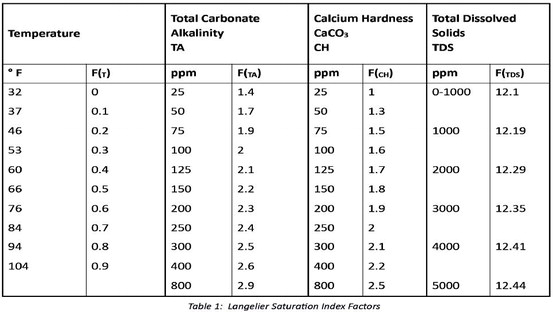Posted on

# So, for those who are ….So, for those who are curious, the following is a discussion about the Langelier Saturation Index.

The LSI provides the mathematical relationship between whether the water might be damaging to surfaces and equipment as a function of the pH, total alkalinity, calcium hardness, temperature and total dissolved solids.

To use the Langelier Saturation Index, the following formula is applied to the water balance parameters:

SI = pH + F(T) + F(TA) + F(CH) – F(TDS)

Temperature, total alkalinity, calcium hardness and total dissolved solids are all given a factor, as shown in the accompanying chart. The pH value is not given a factor and is used asmeasured at the pool. When the various water parameter factors are used in the formula, calculating zero indicates perfectly balanced water. Negative values below -0.3 indicate the water is corrosive. Positive values greater than +0.5 indicate scale forming conditions. It is better to have slightly positive values than slightly negative values because a small amount of scale provides some protection and is less damaging than corrosion.

When cyanuric acid and or borates are used, an additional adjustment must be made to the LSI. When measuring total alkalinity, one is really interested in carbonate alkalinity because it is high or low carbonate levels that cause corrosion or scaling respectively.

Cyanuric acid increases the total alkalinity of the pool water, but not the carbonate alkalinity, which is the alkalinity that matters in water balance.

Borates, which are also sometimes used in swimming pools, have a similar effect.

Total alkalinity is actually a measure of carbonate, bicarbonate, hydroxyl, cyanurate ions and borate ions, if present.

But since neither cyanurate or borate ions play any real role in corrosion or scaling, they should be subtracted out of the measured total alkalinity in order to get a number that is useful for understanding the water’s balance.

In the case of cyanuric acid, this is especially important when the cyanuric acid concentration becomes particularly high. When this occurs, the measured total alkalinity may fall within normal ranges, but the true carbonate alkalinity might be exceedingly low, causing an

CYA and Borates extremely corrosive environment. This circumstance would cause etching to plaster surfaces as well as corroded metals.

In the case of borate use, its effects become more impactful at higher pH values.

CYA correction

At normal pool pH levels, to calculate carbonate alkalinity, the rule of thumb is to subtract out one-third of the cyanuric acid concentration. In other words, total alkalinity is corrected for cyanurate alkalinity by the following equation to yield carbonate alkalinity:

Alkalinity corrected = Alkalinity total – 1/3 Cyanuric Acid

Thus, if the test for total alkalinity shows 100 ppm, while the test for cyanuric acid shows 90, the carbonate alkalinity is roughly 70.

100 – (.33 x 90) = 70

The one-third correction factor is pH dependent. That is because the cyanuric acid and cyanurate ion concentrations are pH dependent. Therefore, it is best to use correction factors that account for this pH dependency.

The cyanuric acid correction factors may be found in the accompanying table.

The borate correction factor is pH dependent. The borate correction factors may be found in the accompanying table. To obtain the carbonate alkalinity from the measured total alkalinity in the presence of borates, the following procedure can be used.

1. Select a borate factor based on the measured pH: F( B)

2. Calculate the carbonate alkalinity from the measured total alkalinity, the measured borate concentration (B), and the selected borate factor (F(B))

Alkalinity corrected = Alkalinity total – (B x F( B))

For example, if the measured total alkalinity is 100 ppm, the pH is 7.6, and the measured borate concentration is 50 ppm, then:

Alkalinity corrected = 100 – (50 x 0.17) Alkalinity corrected = 92 ppm

SI = pH + F( T) + F( TA) + F( CH)

– F( TDS)

However, a calculated carbonate alkalinity must be used prior to selecting F( TA).

For example, consider the following measured water parameters: pH = 7.6; Temperature = 76; Total Alkalinity = 100 ppm; Cyanuric Acid = 90 ppm; Borates = 50 ppm; Calcium Hardness = 200 ppm; Total Dissolved Solids = 800 ppm First, calculate the carbonate alkalinity, or Alkalinity corrected: The Alkalinity corrected is obtained using the cyanuric acid and borate correction factors and the following formula:

Alkalinity corrected = TA – (CYA X .33) – (B x F( B))

Alkalinity corrected = 100 – (100 x .33) – (50 x 0.17) Alkalinity corrected = 58.5 ppm

Next, find the factors for the Langelier Saturation Index from the accompanying table and simply plug them into the general formula:

LSI =

pH + F( T) + F( TA) + F( CH) – F( TDS) LSI = 7.6 + 0.6 + 1.8 + 1.9- 12.1 LSI = -0.2

This number is a little low, but it is still within range.

Table 2: Cyanuric Acid Correction factors as a function of pH

Borate Correction

Putting it all together

As will soon become apparent, calculating the LSI in the presence of cyanuric acid and borates is a bit complicated, which is why many of today’s pool service techs simply install smart phone LSI calculators to save time.

However, for those interested in learning how to calculate the saturation index by hand, the following formula is applied to the water balance parameters:

Table 3 Borate Correction Factors as a function of pHLATEST NEWS